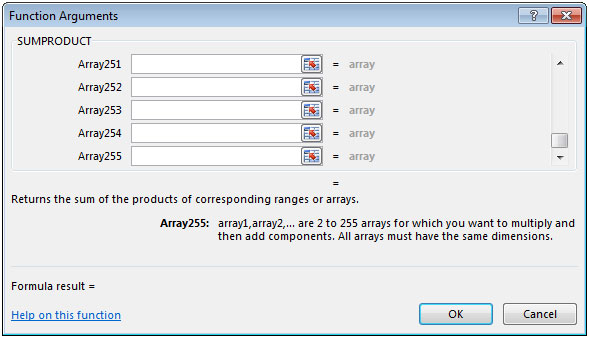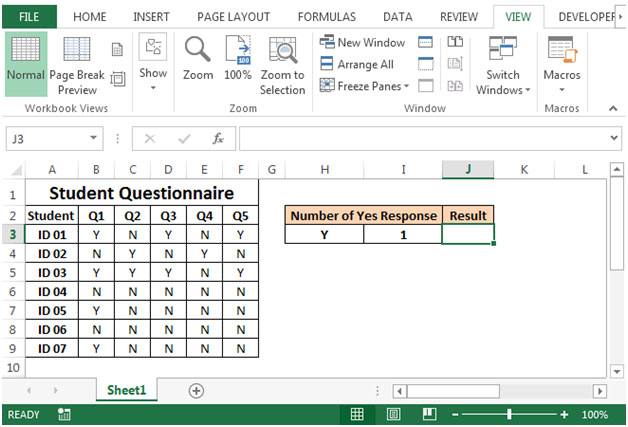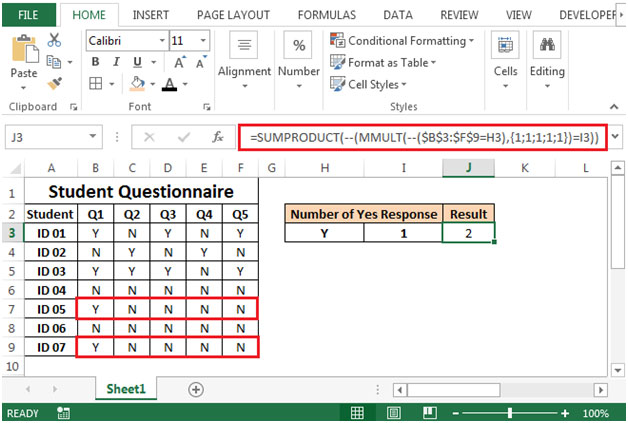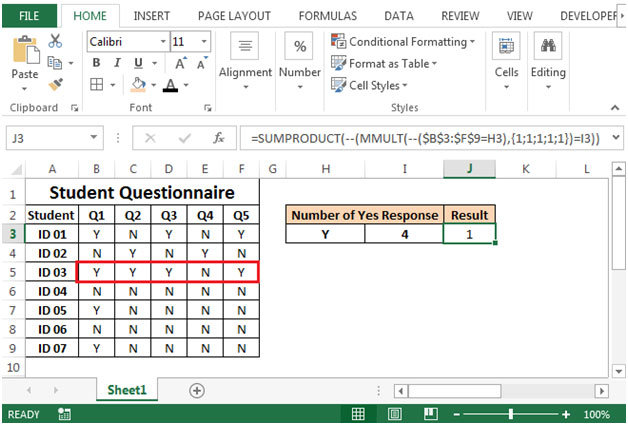# Counting the Number Of Yes Responses in a Student Questionnaire in Excel 2010

In this article, we will learn how to count the number of yes responses in a student questionnaire.

To count the number of Yes responses in a student questionnaire, we will use combination of SUMPRODUCT & MMULT functions to retrieve the output.

SUMPRODUCT: Returns the sum of the products of corresponding ranges or arrays.

Syntax: =SUMPRODUCT(array1,array2,array3,...)

array1,array2,….. array255 are the ranges of cells or arrays that you want to multiply & then add them.

There can be maximum 255 arrays in Sumproduct function. Refer below shown screenshotMMULT: Returns the matrix product of two arrays, an array with the same number of rows as array1 and columns as array2

Syntax: =MMULT(array1,array2)

array1, array2: The arrays you want to multiply.

Let us take an example:

• Column A contains student ID.
• Columns B:F contain the answers (Y/N) to 5 questions from each of 7 students.
• Each row includes the answers of one student.
• We want to count the number of students who gave a certain answer in cell H3 a specific number of times in cell I3.• The formula in cell J3 would be
=SUMPRODUCT(--(MMULT(--(\$B\$3:\$F\$9=H3),{1;1;1;1;1})=I3))• If we change the criteria in cell I3 from 1 to 4 then the formula will update the result accordingly.Terms and Conditions of use

The applications/code on this site are distributed as is and without warranties or liability. In no event shall the owner of the copyrights, or the authors of the applications/code be liable for any loss of profit, any problems or any damage resulting from the use or evaluation of the applications/code.